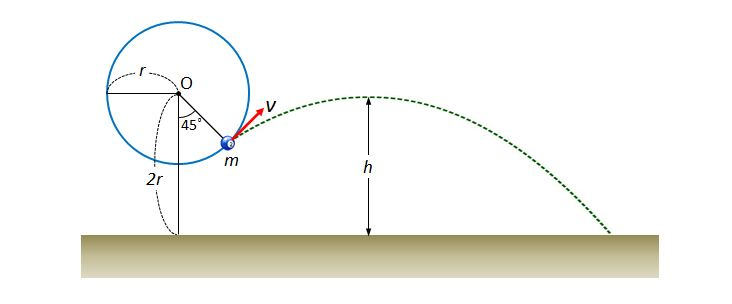# Projectile motion of ball 2A ball tied to the end of a string is in a uniform circular motion at a speed of $v,$ along the circular path with radius $r$ centered at point $O,$ as shown in the above diagram. The height of $O$ from the ground is $2r.$ Suppose that, when the angle between the string and the line drawn from $O$ perpendicularly to the ground is $45°,$ the string is broken loose and the ball flies as indicated by the green dotted line. What is the highest height, $h,$ of the ball during the flight?

$g$ denotes gravitational acceleration.

×